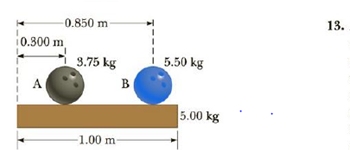Chapter 8, Problem 9P

Chapter
Section
Textbook Problem

Two bowling balls are at rest on top of a uniform wooden plank with their centers of mass located as in Figure P8.9. The plank has a mass of 5.00 kg and is 1.00 m long. Find the horizontal distance from the left end of the plank to the center of mass of the plank-bowling balls system.Figure P8.9

To determine
The center of mass of the plank-bowling ball system.

Explanation
Assume the left end of the object is at the origin of the co-ordinate x .

Given info: The mass of the plank is 5.00kg , mass of the left bowling ball is 3.75kg , mass of the right bowling ball is 5.50kg , the length of the plank is 1.0m , the location of the center mass of the left bowling ball is 0.300m , and the location of the center of mass of the right bowling ball is 0.850m .

The formula for the center of mass of the plank-bowling ball system is,

xcm=mpxp+mB1xB1+mB2xB2mp+mB1+mB2

• mp,m1 , and m2 are masses plank, left, and right end bowling balls.
• xp,xB1 , and xB2 are locations of masses.

Substitute 5.00kg for mp , 0.300kg for mB1 , 5

Still sussing out bartleby?

Check out a sample textbook solution.

See a sample solution

The Solution to Your Study Problems

Bartleby provides explanations to thousands of textbook problems written by our experts, many with advanced degrees!

Get Started